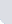TurkZeka 2007/1 Puzzle WettbewerbPUZZLE 10: DIGITAL OPTIMIZATION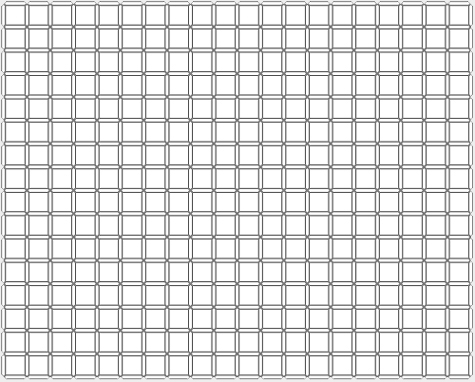By locating the digital numbers below to the above digital platform try to create a closed way which will have as much as corner, length and intersect as it is seen in the example at right.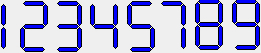THE RULES:

1) You can rotate the numbers as you wish but you can not use their mirror views.

2) The numbers can intersect each other but they cannot overlap each other. In other words, a digit cannot be a digit of two different numbers at the same time.

3) All digits of a number do not have to belong to the same closed way. For instance, four of the five digits forming the number 3 can belong a closed way, and remaining one can belong a different closed way. This situation led you to get bonus. (Look at the Sample Solution 3!) But, one digit of a number cannot be piece of the two different ways at the same time. (Look at the invalid solution 2!)

4) All the ways must be closed. There shouldn�t remain any line out of the closed ways in the platform. (Look at the invalid solution 1!)

 INVALID SOLUTION  1 INVALID SOLUTION  2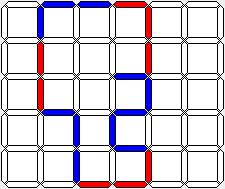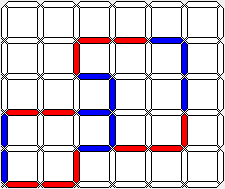5) The lines forming different ways can also intersect each other. However, any line cannot touch each other even at the corners. (Except intersecting point)

6) While creating each closed way you have to use each of the 8 numbers at least once.

Note: This rule is not obeyed in the examples and the sample solutions because of the used small  diagrams.

7) When you create more than one way, having a rule-error at any of the ways will result in not getting point from the others also whether they are correct or not.

8) To get point, there must be at least one intersection in the whole solution. (not on each way!)

9) In your solution, pay attention to show the numbers with different colours which touch or intersect each other.

SCORE CALCULATION :
Firstly; calculate the value of each closed way you create:

To find the value of a way, multiply the sum of the value of the numbers creating a closed way  with the count of the digits creating that way and with the count of the corners on that way.

When the situation which is stated at Rule 3 occurs, namely, if a number is piece of two different closed ways at the same time, the value of that number is calculated for both of two ways separately. Pay attention to the score calculation of sample solution 3.

Then sum up all the values of the ways you had created and multiply this total sum with the total count of all the intersections in the diagram.

 SAMPLE SOLUTION 1 SAMPLE SOLUTION 2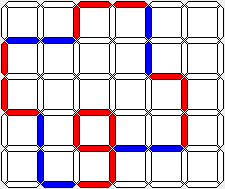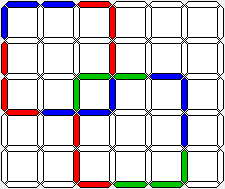Way: 7+1+7+1+9+7+7+1=4040x24x14=13440 Score: 13440x1=13440 1.Way: 7+7+7+7=28 28x12x4=1344 2.Way: 7+7+7+7=28 28x12x4=1344 Score: 1344+1344x2=5376 SAMPLE SOLUTION 3 SAMPLE SOLUTION 4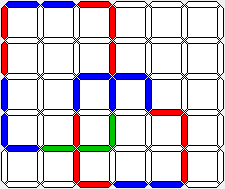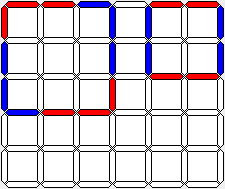1.Way: 1+7+3+7+7+1=2626x14x4=1456 2.Way: 3+7+1+7=1818x12x6=1296 Score: 1456+1296x2=5504 1.Way: 7+7+7+7=2828x12x4=1344 2.Way: 1+1+1+1=44x8x4=128 Score: 1344+128x0=0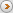You can download the the digital platform in real size by clicking here